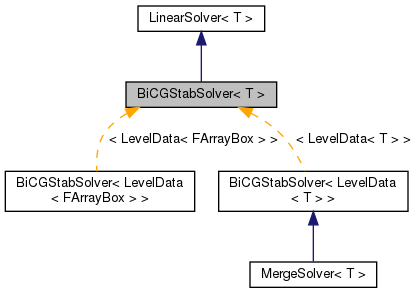Chombo + EB + MF  3.2
BiCGStabSolver< T > Class Template Reference

`#include <BiCGStabSolver.H>`

Inheritance diagram for BiCGStabSolver< T >:[legend]

## Public Member Functions

BiCGStabSolver ()

virtual ~BiCGStabSolver ()

virtual void setHomogeneous (bool a_homogeneous)

virtual void define (LinearOp< T > *a_op, bool a_homogeneous)

virtual void solve (T &a_phi, const T &a_rhs)
solve the equation. More...

virtual void setConvergenceMetrics (Real a_metric, Real a_tolerance)
Set a convergence metric, along with solver tolerance, if desired. More...Public Member Functions inherited from LinearSolver< T >
virtual ~LinearSolver ()

## Public Attributes

bool m_homogeneous

LinearOp< T > * m_op

int m_imax

int m_verbosity

Real m_eps

Real m_reps

Real m_convergenceMetric

Real m_hang

int m_exitStatus

int m_iter

Real m_residual

Real m_initial_residual

Real m_small

int m_numRestarts

int m_normType

## Detailed Description

### template<class T> class BiCGStabSolver< T >

Elliptic solver using the BiCGStab algorithm.

## ◆ BiCGStabSolver()

template<class T >
 BiCGStabSolver< T >::BiCGStabSolver ( )

## ◆ ~BiCGStabSolver()

template<class T >
 BiCGStabSolver< T >::~BiCGStabSolver ( )
virtual

## ◆ setHomogeneous()

template<class T>
 virtual void BiCGStabSolver< T >::setHomogeneous ( bool a_homogeneous )
inlinevirtual

reset whether the solver is homogeneous.

Implements LinearSolver< T >.

Reimplemented in MergeSolver< T >.

## ◆ define()

template<class T>
 void BiCGStabSolver< T >::define ( LinearOp< T > * a_op, bool a_homogeneous )
virtual

define the solver. a_op is the linear operator. a_homogeneous is whether the solver uses homogeneous boundary conditions.

Implements LinearSolver< T >.

Reimplemented in MergeSolver< T >.

Referenced by BiCGStabSolver< LevelData< T > >::setHomogeneous().

## ◆ solve()

template<class T>
 void BiCGStabSolver< T >::solve ( T & a_phi, const T & a_rhs )
virtual

solve the equation.

Implements LinearSolver< T >.

Reimplemented in MergeSolver< T >.

Referenced by BiCGStabSolver< LevelData< T > >::setHomogeneous().

## ◆ setConvergenceMetrics()

template<class T >
 void BiCGStabSolver< T >::setConvergenceMetrics ( Real a_metric, Real a_tolerance )
virtual

Set a convergence metric, along with solver tolerance, if desired.

Default implementation does nothing, since there are probably cases (liked direct solves), where this has no real meaning.

Reimplemented from LinearSolver< T >.

Reimplemented in MergeSolver< T >.

Referenced by BiCGStabSolver< LevelData< T > >::setHomogeneous().

## ◆ m_homogeneous

template<class T>
 bool BiCGStabSolver< T >::m_homogeneous

public member data: whether the solver is restricted to homogeneous boundary conditions

## ◆ m_op

template<class T>
 LinearOp* BiCGStabSolver< T >::m_op

public member data: operator to solve.

## ◆ m_imax

template<class T>
 int BiCGStabSolver< T >::m_imax

public member data: maximum number of iterations

Referenced by BiCGStabSolver< LevelData< T > >::solve().

## ◆ m_verbosity

template<class T>
 int BiCGStabSolver< T >::m_verbosity

public member data: how much screen out put the user wants. set = 0 for no output.

Referenced by MultilevelLinearOp< T >::define(), and BiCGStabSolver< LevelData< T > >::solve().

## ◆ m_eps

template<class T>
 Real BiCGStabSolver< T >::m_eps

public member data: solver tolerance

## ◆ m_reps

template<class T>
 Real BiCGStabSolver< T >::m_reps

public member data: relative solver tolerance

Referenced by BiCGStabSolver< LevelData< T > >::solve().

## ◆ m_convergenceMetric

template<class T>
 Real BiCGStabSolver< T >::m_convergenceMetric

public member data: solver convergence metric – if negative, use initial residual; if positive, then use m_convergenceMetric

## ◆ m_hang

template<class T>
 Real BiCGStabSolver< T >::m_hang

public member data: minium norm of solution should change per iterations

Referenced by BiCGStabSolver< LevelData< T > >::solve().

## ◆ m_exitStatus

template<class T>
 int BiCGStabSolver< T >::m_exitStatus

public member data: set = -1 if solver exited for an unknown reason set = 1 if solver converged to tolerance set = 2 if rho = 0 set = 3 if max number of restarts was reached

Referenced by BiCGStabSolver< LevelData< T > >::solve().

## ◆ m_iter

template<class T>
 int BiCGStabSolver< T >::m_iter

public member data: iteration number. This is a member so that it can be queried after a solve, for example.

Referenced by BiCGStabSolver< LevelData< T > >::solve().

## ◆ m_residual

template<class T>
 Real BiCGStabSolver< T >::m_residual

public member data: current residual norm. This is a member so that it can be queried after a solve, for example

Referenced by BiCGStabSolver< LevelData< T > >::solve().

## ◆ m_initial_residual

template<class T>
 Real BiCGStabSolver< T >::m_initial_residual

public member data: initial residual norm. This is a member so that it can be queried after a solve, for example

Referenced by BiCGStabSolver< LevelData< T > >::solve().

## ◆ m_small

template<class T>
 Real BiCGStabSolver< T >::m_small

public member data: what the algorithm should consider "close to zero"

Referenced by BiCGStabSolver< LevelData< T > >::solve().

## ◆ m_numRestarts

template<class T>
 int BiCGStabSolver< T >::m_numRestarts

public member data: number of times the algorithm can restart

Referenced by BiCGStabSolver< LevelData< T > >::solve().

## ◆ m_normType

template<class T>
 int BiCGStabSolver< T >::m_normType

public member data: norm to be used when evaluation convergence. 0 is max norm, 1 is L(1), 2 is L(2) and so on.

Referenced by BiCGStabSolver< LevelData< T > >::solve().

The documentation for this class was generated from the following file: